# Prometheus 数据存储那些事儿

## 概述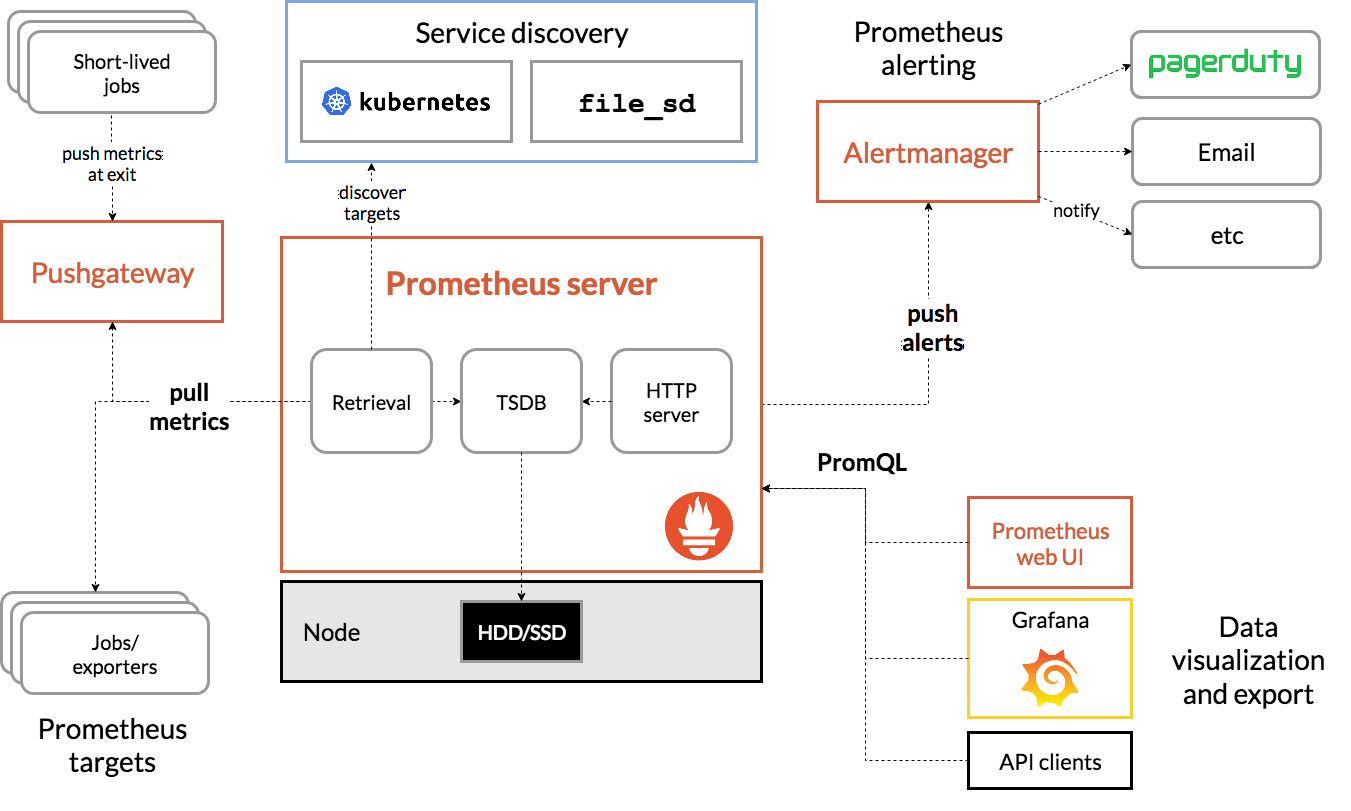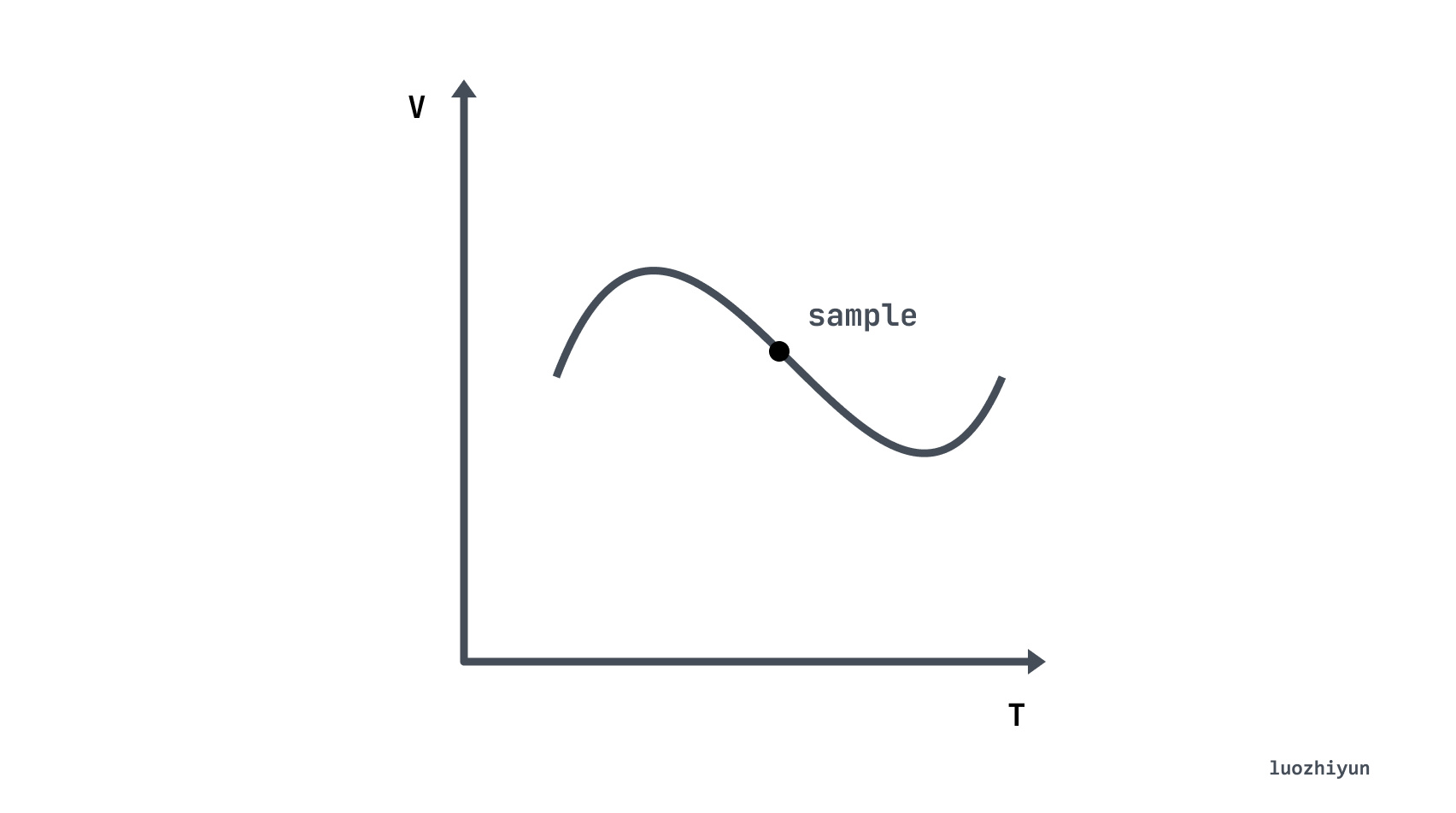``````  ^
│   . . . . . . . . . . . . . . . . .   . .   node_cpu{cpu="cpu0",mode="idle"}
│     . . . . . . . . . . . . . . . . . . .   node_cpu{cpu="cpu0",mode="system"}
│     . . . . . . . . . .   . . . . . . . .   node_load1{}
│     . . . . . . . . . . . . . . . .   . .
v
<------------------ 时间 ---------------->``````

• 指标（metric name）：metric name和描述当前样本特。例如上面的 node_cpu 表示节点的 CPU 信息；

• 时间戳（timestamp）：一个精确到毫秒的时间戳，表示数据记录的时间；

• 标签（Lables）：使同一个时间序列有了不同维度的识别，例如 `node_cpu{cpu="cpu0",mode="idle"}` 表示所有 cpu0 状态为 idle 的信息，当 mode 等于 system 时则为一个新的 metric；

• 样本值（value）： 一个 float64 的浮点型数据表示当前样本的值，也就是上图的 Y 轴。

## TSDB 数据写入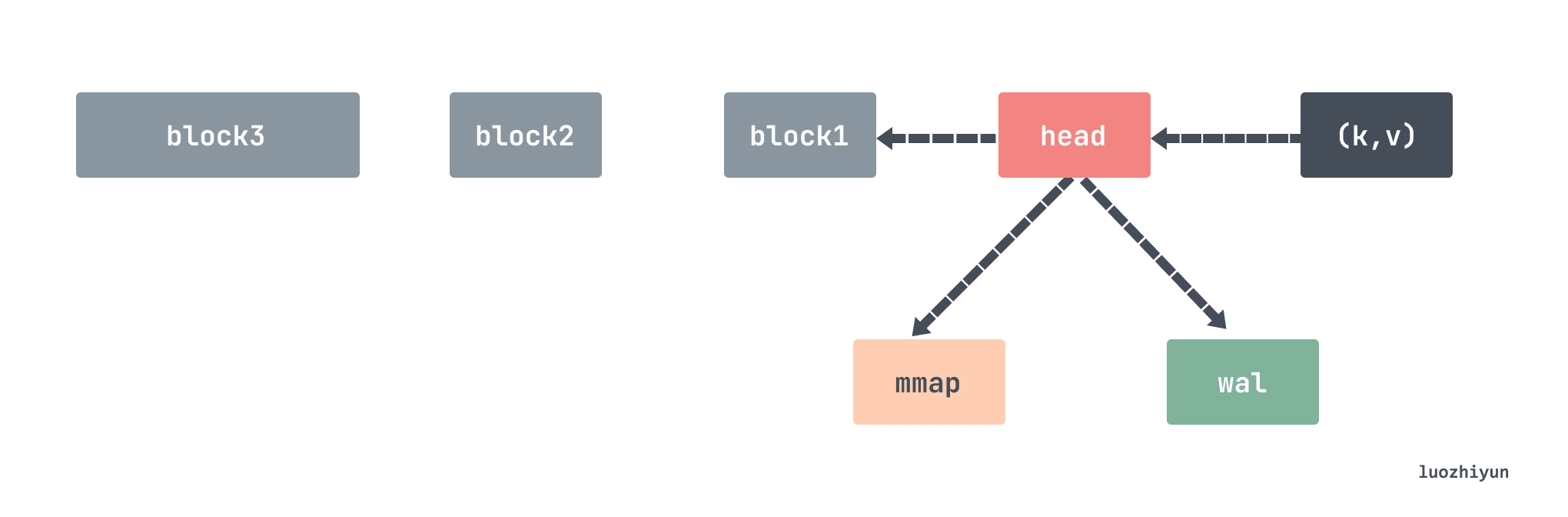``````./data
├── 01GJ9EKDWSS1TA1V0RBP707V21
│   ├── chunks
│   │   └── 000001
│   ├── index
│   ├── meta.json
│   └── tombstones
│   └── 000012
└── wal
├── 00000013
├── 00000014
├── 00000015
└── checkpoint.00000012
└── 00000000``````

wal 文件夹里面存放的数据是当前正在写入的数据，里面包含多个数据段文件，一个文件默认最大 128M，Prometheus 会至少保留3个文件，对于高负载的机器会至少保留2小时的数据。wal 文件夹里面的数据是没有压缩过的，所以会比 block 里面的数据略大一些。

v2.19之前，最近 2 小时的指标数据存储在内存中，v2.19 引入 head block，最近的指标数据存储在内存中，当内存存满时将数据刷入到磁盘中，并通过一个引用关联刷到磁盘的数据。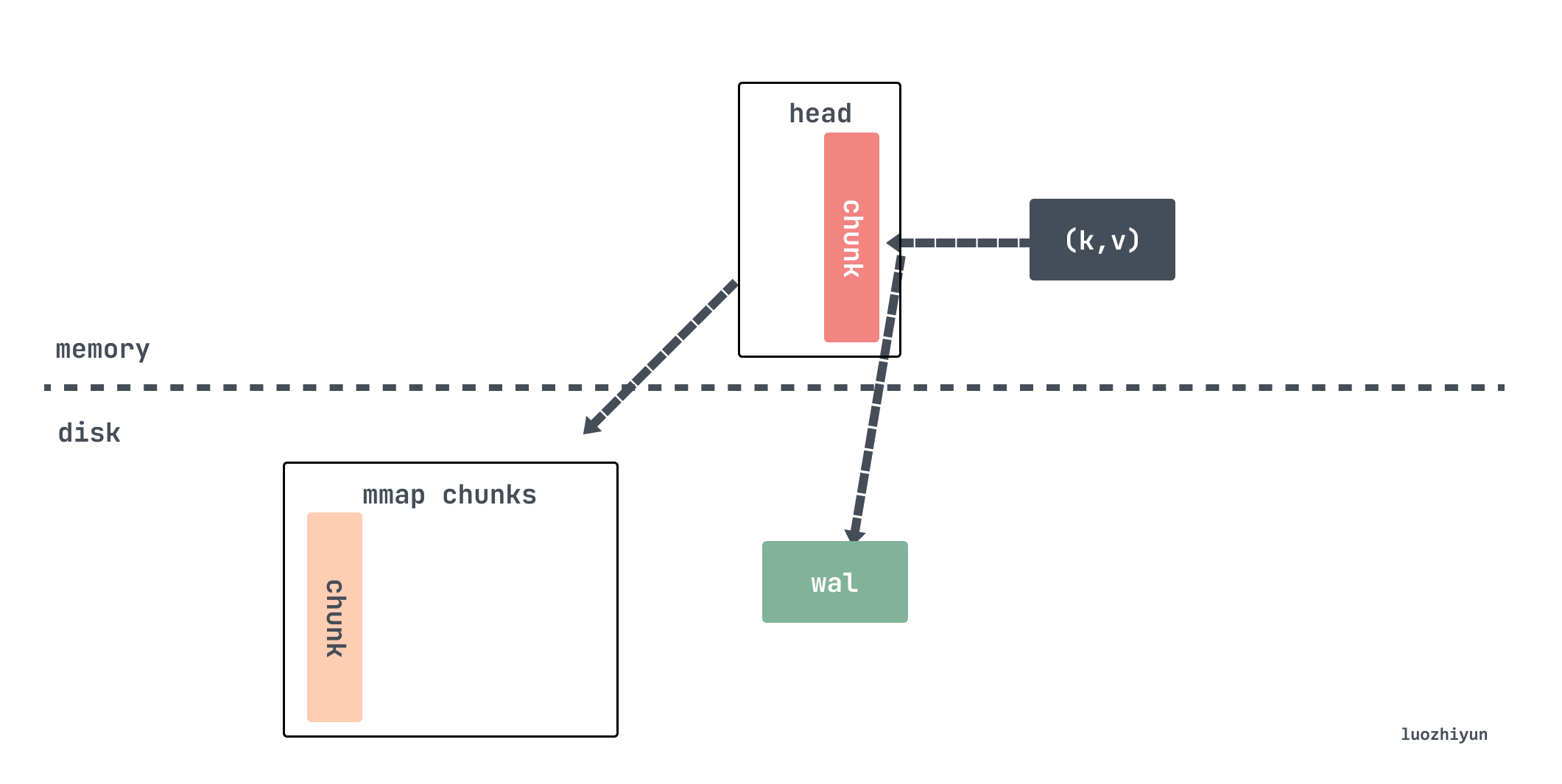head block 是唯一活跃的 block，除了它以外，其它 blocks 都是不可变的。我们在上面也说了，数据每次从 scraper 抓取过来之后都会存放到 appender 里面，这个 appender 实际上就是 headAppender， 通过调用 Append 方法将数据暂时缓存起来，通过appender来做批量的添加，然后 commit 才会真正写数据。

``````// tsdb/head_append.go
func (a *headAppender) Append(ref storage.SeriesRef, lset labels.Labels, t int64, v float64) (storage.SeriesRef, error) {
// 获取该stripeseries对应的memSeries，ref实际上是lset的hash值
if s == nil {

var created bool
var err error
// 不存在则创建为该指标创建一个 memSeries
s, created, err = a.head.getOrCreate(lset.Hash(), lset)
if err != nil {
return 0, err
}
if created {
a.series = append(a.series, record.RefSeries{
Ref:    s.ref,
Labels: lset,
})
}
}
...
// 将数据缓存起来
a.samples = append(a.samples, record.RefSample{
Ref: s.ref,
T:   t,
V:   v,
})
a.sampleSeries = append(a.sampleSeries, s)
return storage.SeriesRef(s.ref), nil
}``````

``````// tsdb/head_append.go
func (a *headAppender) Commit() (err error) {

// 写入wal日志
if err := a.log(); err != nil {
_ = a.Rollback() // Most likely the same error will happen again.
return errors.Wrap(err, "write to WAL")
}
...
for i, s := range a.samples {
series = a.sampleSeries[i]
series.Lock()
...
// 写入数据
ok, chunkCreated = series.append(s.T, s.V, a.appendID, a.head.chunkDiskMapper, chunkRange)
series.Unlock()
}
...
return nil
}``````

Commit 方法会将保存在 samples 中的数据拿出来，然后通过调用 memSeries 的 append 将数据循环写入。

``````// tsdb/head_append.go
func (s *memSeries) append(t int64, v float64, appendID uint64, chunkDiskMapper *chunks.ChunkDiskMapper, chunkRange int64) (sampleInOrder, chunkCreated bool) {
c, sampleInOrder, chunkCreated := s.appendPreprocessor(t, chunkenc.EncXOR, chunkDiskMapper, chunkRange)
// 调用 xorAppender 添加指标数据
s.app.Append(t, v)
c.maxTime = t
...
return true, chunkCreated
}``````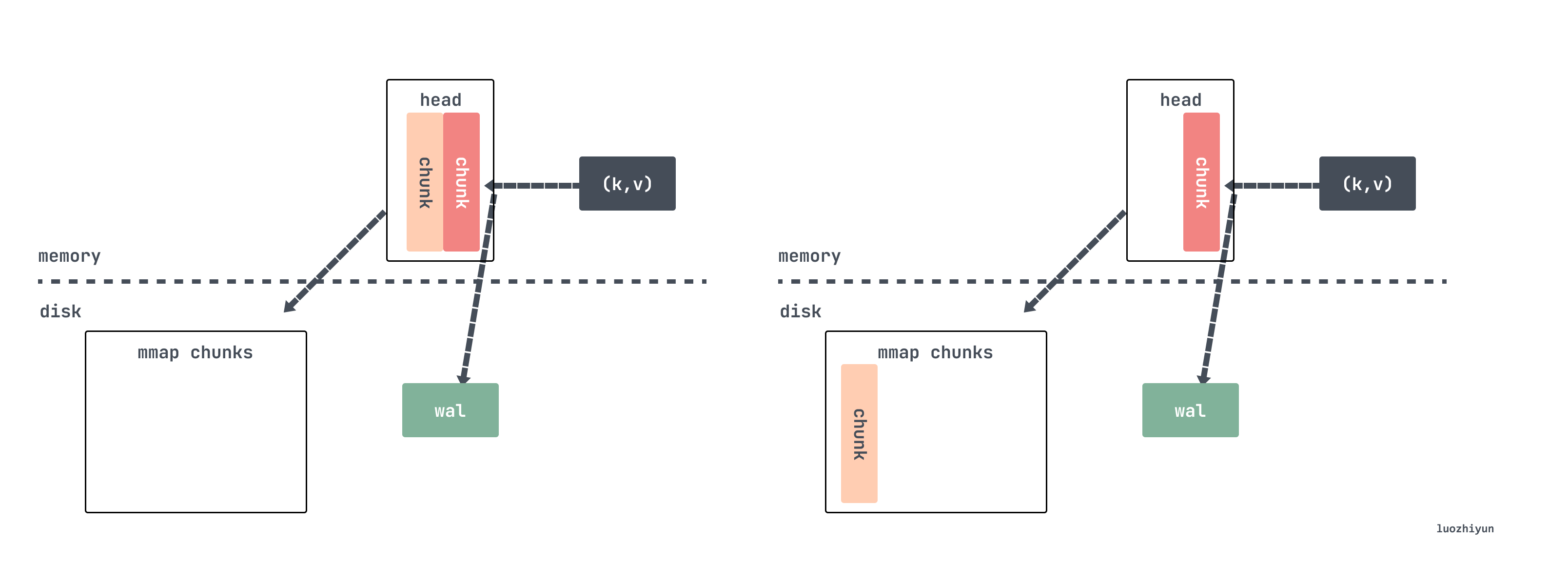``````// tsdb/head_append.go
func (s *memSeries) appendPreprocessor(
t int64, e chunkenc.Encoding, chunkDiskMapper *chunks.ChunkDiskMapper, chunkRange int64,
) (c *memChunk, sampleInOrder, chunkCreated bool) {
const samplesPerChunk = 120

if c == nil {
c = s.cutNewHeadChunk(t, e, chunkDiskMapper, chunkRange)
chunkCreated = true
}

numSamples := c.chunk.NumSamples()
if numSamples == 0 {
c.minTime = t
// chunkRange 默认是2hour，这里算的下次开始的时间是个以2为倍数的整数时间
s.nextAt = rangeForTimestamp(c.minTime, chunkRange)
}
// 到1/4时，重新计算预估nextAt，下一个chunk的时间
if numSamples == samplesPerChunk/4 {
s.nextAt = computeChunkEndTime(c.minTime, c.maxTime, s.nextAt)
}
if t >= s.nextAt || numSamples >= samplesPerChunk*2 {
c = s.cutNewHeadChunk(t, e, chunkDiskMapper, chunkRange)
chunkCreated = true
}

return c, true, chunkCreated
}``````

``````const MaxHeadChunkFileSize = 128 * 1024 * 1024 // 128 MiB.
func (f *chunkPos) shouldCutNewFile(bytesToWrite uint64) bool {
return f.offset == 0 || // First head chunk file.
}``````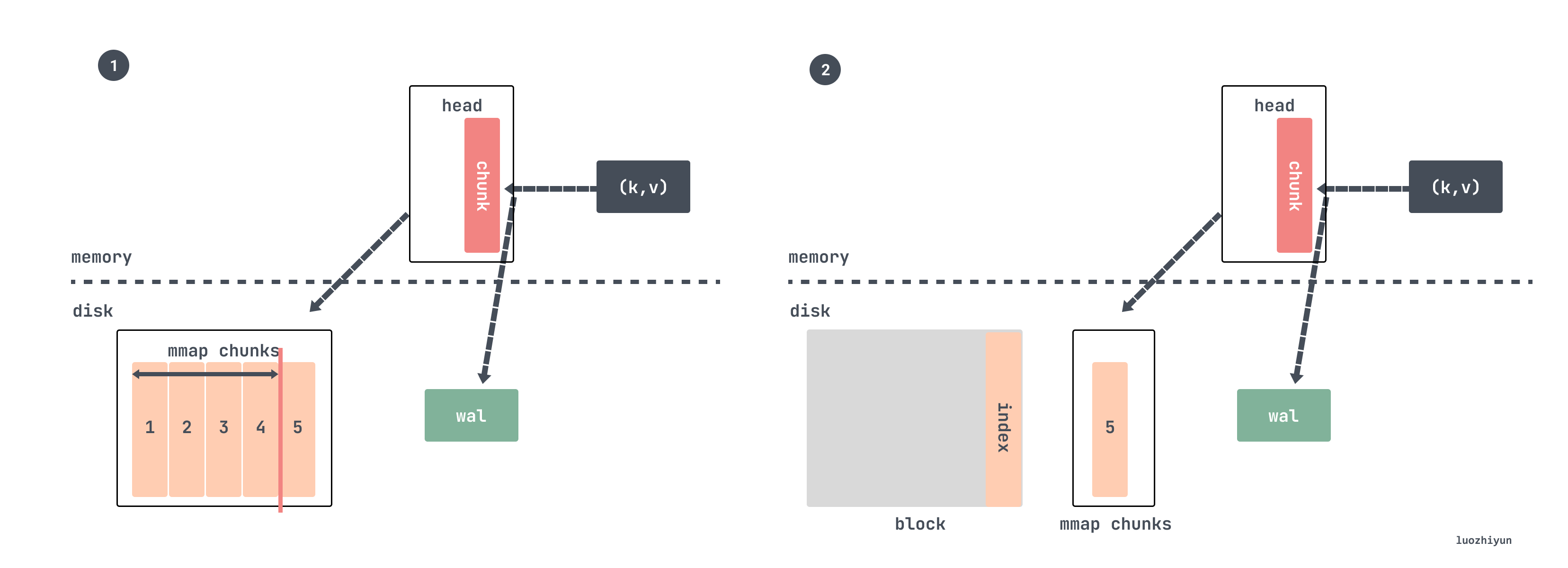tsdb 在初始化的时候会后台运行一个 goroutine，每分钟检查一下 chuan的 chunkRange 跨度是否大于 `chunkRange*3/2`

``````//tsdb/db.go
func (db *DB) run() {
for {
select {
case <-time.After(1 * time.Minute):
select {
case db.compactc <- struct{}{}:
default:
}
case <-db.compactc:
// 校验是否进行压缩
err := db.Compact()
...
case <-db.stopc:
return
}
}
}``````

Compact 方法里面会根据调用 compactable 方法进行校验：

``````func (h *Head) compactable() bool {
}``````

chunkRange 默认值是 DefaultBlockDuration 为 2 小时：

``DefaultBlockDuration = int64(2 * time.Hour / time.Millisecond)``

``````//tsdb/db.go
// 最小数据时间
// 最大数据时间

func rangeForTimestamp(t, width int64) (maxt int64) {
// width 为2小时
return (t/width)*width + width
}``````

### wal

wal 是一个日志序列用来记录数据库发生的一些操作，每次在写入、修改、删除之前就会先记录一条在 wal 日志里。主要作用就是在程序挂了之后还能用这份日志数据来做恢复用，因为我们前面也说了，head 里面的chunk 并没有持久化。

wal 按序列号依次递增存储在 wal 文件夹里面，每个文件被称为 segment 默认 128MB 大小。Prometheus称这样的文件为 Segment，其中存放的就是对内存中 series 以及 sample 数据的备份。

``````data
└── wal
├── checkpoint.000003
|   ├── 000000
|   └── 000001
├── 000004
└── 000005``````

``````//tsdb/head.go
// 获取磁盘的 wal Segments 时间跨度范围
first, last, err := wlog.Segments(h.wal.Dir())
// 重新调整被删除的结束时间，只删除2/3 的数据
last = first + (last-first)*2/3 ``````

``````data
└── wal
├── 000000
├── 000001
├── 000002
├── 000003
├── 000004
└── 000005``````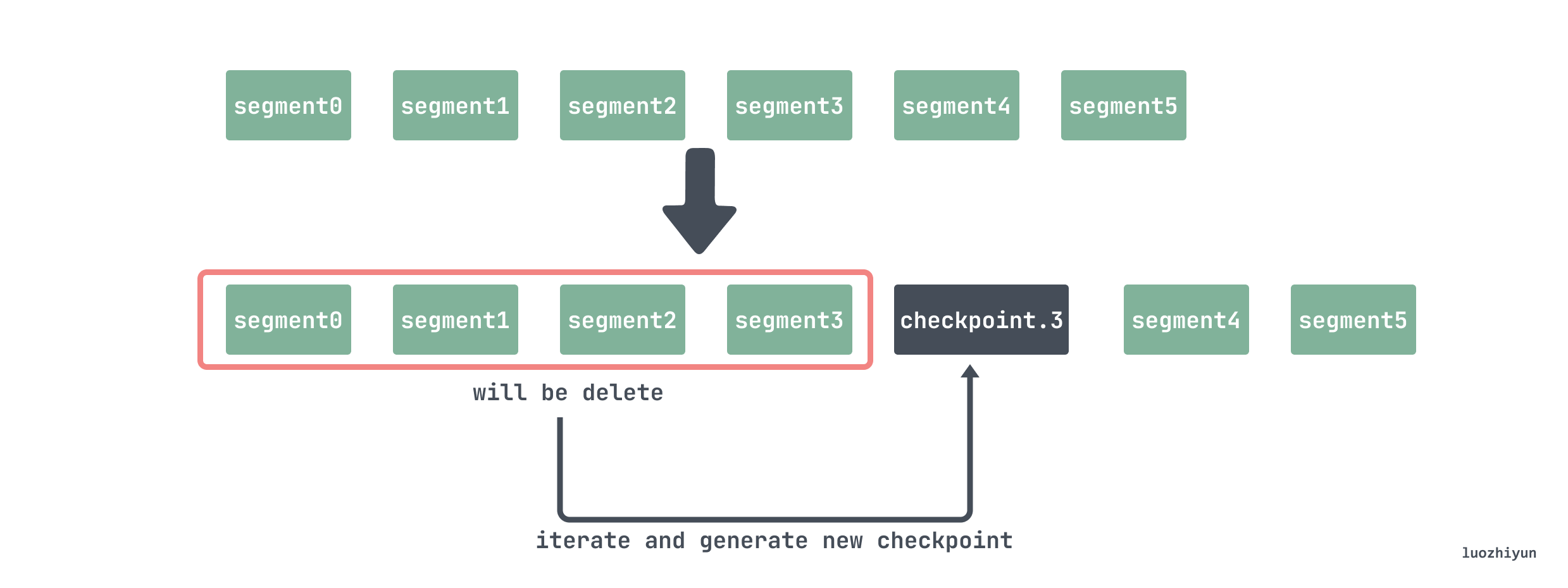``````// tsdb/wlog/checkpoint.go
func Checkpoint(logger log.Logger, w *WL, from, to int, keep func(id chunks.HeadSeriesRef) bool, mint int64) (*CheckpointStats, error) {
...
var (
buf              []byte
recs             [][]byte
)
...
for r.Next() {
// buf 起始位置
start := len(buf)
//读出数据
rec := r.Record()

switch dec.Type(rec) {
case record.Series:
series, err = dec.Series(rec, series)
// Drop irrelevant series in place.
repl := series[:0]
for _, s := range series {
// 校验该 series 是否还存在在 head 中
if keep(s.Ref) {
//保留
repl = append(repl, s)
}
}
if len(repl) > 0 { // 将要保留的数据写入到 buffer 中
buf = enc.Series(repl, buf)
}
stats.TotalSeries += len(series)
stats.DroppedSeries += len(series) - len(repl)
...
case record.Samples:
samples, err = dec.Samples(rec, samples)
repl := samples[:0]
for _, s := range samples {
// 校验该sample的时间是否在mint之后
if s.T >= mint {
//之后的数据需要保留
repl = append(repl, s)
}
}
if len(repl) > 0 {// 将要保留的数据写入到 buffer 中
buf = enc.Samples(repl, buf)
}
stats.TotalSamples += len(samples)
stats.DroppedSamples += len(samples) - len(repl)
...
// 将buf数据写入到recs中
recs = append(recs, buf[start:])
// 如果buf 中的数据已经超过 1M，需要将数据写入到 checkpoint中
if len(buf) > 1*1024*1024 {
if err := cp.Log(recs...); err != nil {
return nil, errors.Wrap(err, "flush records")
}
buf, recs = buf[:0], recs[:0]
}
}
// 遍历完之后将残余数据checkpoint中
if err := cp.Log(recs...); err != nil {
return nil, errors.Wrap(err, "flush records")
}
}``````

### block

``````./data
├── 01BKGTZQ1SYQJTR4PB43C8PD98
│   ├── chunks
│   │   └── 000001
│   ├── tombstones
│   ├── index
│   └── meta.json``````
• meta.json 元信息，主要包括 block 的 minTime/maxTime，方便查询时过滤；
• chunks 是一个子目录，包含了若干个从 000001 开始编号的文件，一般每个文件大小的上限为512M。文件中存储的就是在时间窗口[minTime，maxTime]以内的所有 samples；
• tombstones 用于存储对于 series 的删除记录。如果删除了某个时间序列，Prometheus并不会立即对它进行清理，而是会在 tombstones 做一次记录，等到下一次Block压缩合并的时候统一清理；
• index 索引文件，主要包含倒排索引的信息；

#### index 数据查找

``````┌────────────────────────────┬─────────────────────┐
│ magic(0xBAAAD700) <4b>     │ version(1) <1 byte> │
├────────────────────────────┴─────────────────────┤
│ ┌──────────────────────────────────────────────┐ │
│ │                 Symbol Table                 │ │
│ ├──────────────────────────────────────────────┤ │
│ │                    Series                    │ │
│ ├──────────────────────────────────────────────┤ │
│ │                 Label Index 1                │ │
│ ├──────────────────────────────────────────────┤ │
│ │                      ...                     │ │
│ ├──────────────────────────────────────────────┤ │
│ │                 Label Index N                │ │
│ ├──────────────────────────────────────────────┤ │
│ │                   Postings 1                 │ │
│ ├──────────────────────────────────────────────┤ │
│ │                      ...                     │ │
│ ├──────────────────────────────────────────────┤ │
│ │                   Postings N                 │ │
│ ├──────────────────────────────────────────────┤ │
│ │               Label Index Table              │ │
│ ├──────────────────────────────────────────────┤ │
│ │                 Postings Table               │ │
│ ├──────────────────────────────────────────────┤ │
│ │                      TOC                     │ │
│ └──────────────────────────────────────────────┘ │
└──────────────────────────────────────────────────┘``````

``````┌─────────────────────────────────────────┐
│ ref(symbols) <8b>                       │
├─────────────────────────────────────────┤
│ ref(series) <8b>                        │
├─────────────────────────────────────────┤
│ ref(label indices start) <8b>           │
├─────────────────────────────────────────┤
│ ref(label offset table) <8b>            │
├─────────────────────────────────────────┤
│ ref(postings start) <8b>                │
├─────────────────────────────────────────┤
│ ref(postings offset table) <8b>         │
├─────────────────────────────────────────┤
│ CRC32 <4b>                              │
└─────────────────────────────────────────┘``````

• 首先给每个 series 分配1个 id，用 seriesId 查询 series ，这是前向索引，查询时间复杂度=O(1)；
• 构造 label 的索引，若`seriesId={2,5,10,29}`都含有`label: app='nginx'`，那么，对于`app='nginx"`, `{2,5,10,29}`就是它的倒排索引；``````┌──────────────────────────────────────────────────────────────────────────┐
│ len <uvarint>                                                            │
├──────────────────────────────────────────────────────────────────────────┤
│ ┌──────────────────────────────────────────────────────────────────────┐ │
│ │                     labels count <uvarint64>  //lable数量             │ │
│ ├──────────────────────────────────────────────────────────────────────┤ │
│ │ lable列表     ┌────────────────────────────────────────────┐          │ │
│ │              │ ref(l_i.name) <uvarint32>   //label名      │          │ │
│ │              ├────────────────────────────────────────────┤          │ │
│ │              │ ref(l_i.value) <uvarint32>  //label值      │          │ │
│ │              └────────────────────────────────────────────┘          │ │
│ │                             ...                                      │ │
│ ├──────────────────────────────────────────────────────────────────────┤ │
│ │                     chunks count <uvarint64>  //chunk数量             │ │
│ ├──────────────────────────────────────────────────────────────────────┤ │
│ │ chunk列表     ┌────────────────────────────────────────────┐          │ │
│ │              │ c_0.mint <varint64>                        │          │ │
│ │              ├────────────────────────────────────────────┤          │ │
│ │              │ c_0.maxt - c_0.mint <uvarint64>            │          │ │
│ │              ├────────────────────────────────────────────┤          │ │
│ │              │ ref(c_0.data) <uvarint64>                  │          │ │
│ │              └────────────────────────────────────────────┘          │ │
│ │              ┌────────────────────────────────────────────┐          │ │
│ │              │ c_i.mint - c_i-1.maxt <uvarint64>          │          │ │
│ │              ├────────────────────────────────────────────┤          │ │
│ │              │ c_i.maxt - c_i.mint <uvarint64>            │          │ │
│ │              ├────────────────────────────────────────────┤          │ │
│ │              │ ref(c_i.data) - ref(c_i-1.data) <varint64> │          │ │
│ │              └────────────────────────────────────────────┘          │ │
│ │                             ...                                      │ │
│ └──────────────────────────────────────────────────────────────────────┘ │
├──────────────────────────────────────────────────────────────────────────┤
│ CRC32 <4b>                                                               │
└──────────────────────────────────────────────────────────────────────────┘``````

## Reference

https://tech.ipalfish.com/blog/2020/03/31/the-evolution-of-prometheus-storage-layer/

https://github.com/prometheus/prometheus/blob/main/tsdb/docs/format/index.md

https://liujiacai.net/blog/2021/04/11/prometheus-storage-engine/

Be smarter in how we look at matchers https://github.com/prometheus-junkyard/tsdb/pull/572

https://prometheus.kpingfan.com/

https://yunlzheng.gitbook.io/prometheus-book/

https://prometheus.io/docs/prometheus/latest/storage/

https://grafana.com/blog/2022/09/07/new-in-grafana-mimir-introducing-out-of-order-sample-ingestion/

https://github.com/prometheus/prometheus/blob/release-2.40/tsdb/docs/refs.md

Persistent Block and its Index https://ganeshvernekar.com/blog/prometheus-tsdb-persistent-block-and-its-index

New in Grafana Mimir: Introducing out-of-order sample ingestion https://grafana.com/blog/2022/09/07/new-in-grafana-mimir-introducing-out-of-order-sample-ingestion/?mdm=social

https://segmentfault.com/a/1190000041117609